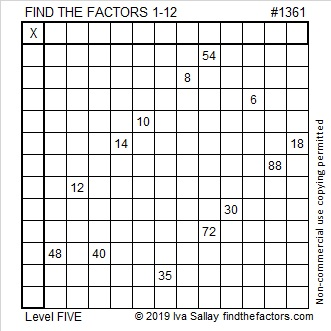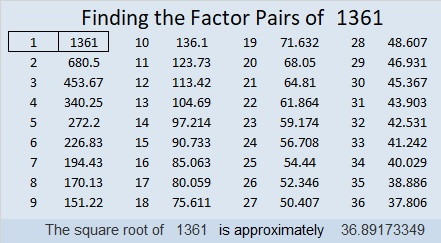# 1361 and Level 5

If you carefully use logic instead of guessing and checking you can find the unique solution to this puzzle without tearing your hair out!Print the puzzles or type the solution in this excel file: 12 Factors 1357-1365

Here are some facts about the number 1361:

• 1361 is a prime number.
• Prime factorization: 1361 is prime.
• 1361 has no exponents greater than 1 in its prime factorization, so √1361 cannot be simplified.
• The exponent in the prime factorization is 1. Adding one to that exponent we get (1 + 1) = 2. Therefore 1361 has exactly 2 factors.
• The factors of 1361 are outlined with their factor pair partners in the graphic below.

How do we know that 1361 is a prime number? If 1361 were not a prime number, then it would be divisible by at least one prime number less than or equal to √1361. Since 1361 cannot be divided evenly by 2, 3, 5, 7, 11, 13, 17, 19, 23, 29 or 31, we know that 1361 is a prime number.1361 is the first prime number after 1327. That was 34 numbers ago!

1361 is the sum of two squares:
31² + 20² = 1361

1361 is the hypotenuse of a Pythagorean triple:
561-1240-1361 calculated from 31² – 20², 2(31)(20), 31² + 20²

Here’s another way we know that 1361 is a prime number: Since its last two digits divided by 4 leave a remainder of 1, and 31² + 20² = 1361 with 31 and 20 having no common prime factors, 1361 will be prime unless it is divisible by a prime number Pythagorean triple hypotenuse less than or equal to √1361. Since 1361 is not divisible by 5, 13, 17, or 29, we know that 1361 is a prime number.

This site uses Akismet to reduce spam. Learn how your comment data is processed.